Computational & Technology Resources an online resource for computational,engineering & technology publications not logged in - login Civil-Comp ProceedingsISSN 1759-3433 CCP: 83PROCEEDINGS OF THE EIGHTH INTERNATIONAL CONFERENCE ON COMPUTATIONAL STRUCTURES TECHNOLOGY Edited by: B.H.V. Topping, G. Montero and R. Montenegro Paper 214Optimality Criterion Methods and Sequential Approximate Optimization in the Classical Topology Layout Problem A.A. Groenwold1 and L.F.P. Etman21Department of Mechanical Engineering, University of Stellenbosch, South Africa 2Department of Mechanical Engineering, Eindhoven University of Technology, The Netherlands doi:10.4203/ccp.83.214 Full Bibliographic Reference for this paper A.A. Groenwold, L.F.P. Etman, "Optimality Criterion Methods and Sequential Approximate Optimization in the Classical Topology Layout Problem", in B.H.V. Topping, G. Montero, R. Montenegro, (Editors), "Proceedings of the Eighth International Conference on Computational Structures Technology", Civil-Comp Press, Stirlingshire, UK, Paper 214, 2006. doi:10.4203/ccp.83.214 Keywords: topology optimization, optimality criterion, sequential approximate optimization, incomplete series expansion, duality, exponential intervening variables. Summary In this paper, we study the 'classical' topology optimization problem, in which minimum compliance is sought, subject to a single linear constraint on volume. We use the simplest strategy for the introduction of topological features into a structure, being the popular simple isotropic material with penalization (SIMP) method, independently proposed by Bendsøe  and Rozvany and Zhou . In solving the classical topology optimization problem, the well-known optimality criterion (OC) method is often used, being an expression of the Karush-Kuhn-Tucker stationary conditions at optimality of the Lagrangian of the classical topology optimization problem. Unfortunately, direct application of the OC to the classical topology optimization problem results in severe oscillatory behavior; hence the OC statement is often augmented by a heuristic scheme for updating the design variables and the single Lagrangian multiplier iteratively. As an alternative to the OC methods, sequential approximate optimization (SAO) methods are also often used in topology optimization, in particular when more than one constraint is considered (since OC methods become cumbersome). In turn, the SAO algorithms are often based on strictly convex and separable primal approximate subproblems, which may be transformed into highly efficient dual subproblems when the number of constraints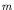are far less than the number of design variables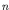. Examples of successfull SAO algorithms are, amongst others, the well-known CONLIN algorithm  and its generalization, the method of moving asymptotes (MMA) [4,5]. Both algorithms depend on the sequential solution of strictly convex and separable approximate subproblems, and in both CONLIN and MMA, the approximate subproblems contain reciprocal intervening (intermediate) variables, substituted into the linear first order Taylor series expansion. In this study, we propose strictly convex subproblems for use in SAO algorithms, using duality. Rather than using only the customary reciprocal intervening variables, we also resort to exponential intervening variables. The subproblems may then be solved in a rudimentary SAO algorithm. We then demonstrate that direct application of the ever-popular optimality criterion to the classical topology optimization problem, combined with the heuristic updating scheme proposed by Bendsøe , is similar to a rudimentary dual SAO algorithm in which the subproblems are based on reciprocal intervening variables. The rigorous dual algorithmic development however does not require heuristics like numerical damping coefficients in the iterative updating scheme. Instead, the updating scheme follows naturally. In fact, the 'direct' OC method with numerical damping and the SAO algorithm based on reciprocal intervening variables are identical if the numerical damping coefficient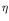in the direct OC method is, as usual, selected as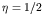. The relationship between dual SAO algorithms and OC methods has previously been demonstrated for the minimum weight optimization problem (i.e. for problems with a linear objective function), but seemingly, not for the topology problem. In fact, Bendsøe  remarks that the 'direct' OC method in topology optimization is reminiscent of the fully stressed design (FSD) problem. Finally, we show that the use of exponential intervening variables results in a dual formulation of superior efficiency and accuracy to subproblems based on reciprocal intervening variables (and hence, the OC method with the numerical damping coefficient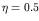hitherto used), at very little added complexity. Computational experiments confirm the efficiency of our proposed methodology; to this end, comparative results for the method of moving asymptotes (MMA) are presented. References 1 M.P. Bendsøe. Optimal shape design as a material distribution problem. Struct. Optim., 1:193-202, 1989. doi:10.1007/BF01650949 2 G.I.N. Rozvany and M. Zhou. Applications of COC method in layout optimization. In H. Eschenauer, C. Mattheck, and N. Olhoff, editors, Proc. Engineering Optimization in Design Processes, pages 59-70, Berlin, 1991. Springer-Verlag. 3 C. Fleury and V. Braibant. Structural optimization: a new dual method using mixed variables. Int. J. Numer. Meth. Eng., 23:409-428, 1986. doi:10.1002/nme.1620230307 4 K. Svanberg. The method of moving asymptotes - a new method for structural optimization Int. J. Numer. Meth. Eng., 24:359-373, 1987. doi:10.1002/nme.1620240207 5 K. Svanberg. A globally convergent version of MMA without linesearch. In G.I.N. Rozvany and N. Olhoff, editors, Proc. First World Congress on Structural and Multidisciplinary Optimization, pages 9-16, Goslar, Germany, 1995. purchase the full-text of this paper (price £20) Back to top ©Civil-Comp Limited 2022 - terms & conditions